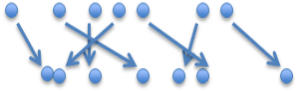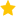Resources for teaching statistics: Key Resources, Teacher Preparation

### What classifies a task as an experiment? (2.10)

There must be an intervention.

Type of experiment: Comparison

It is important to note that an experiment cannot be a prediction-type task involving scatterplots. For example, dropping a ball at various heights, recording the bounce heights and then using these results to predict a bounce height from a drop height.

There are two types of designs to consider for Achievement Standard 2.10:

1.  Two independent group comparison

Design:  There must be random allocation to the two groups in an attempt to make the comparison fair.   Do not use the word “sample”, rather use the word “group”.

The two groups must be independent.

Analysis:   Compare using dot plots and/or boxplots.  Students describe what they see and use the mean, standard deviation, describe variation, clusters etc.

Conclusion:  The conclusion is descriptive.    For example, these data suggest that using a fixed target line resulted in (or caused)the students jumping further on average than when no target line was used.

There are two types of inference:   experiment-to-causal type inference and sample-to-population type inference.   The conclusion statement of an experiment at NZC Level 7 must at least include an experiment-to-causal type inference statement as above.

A sample-to-population type inference only is not acceptable.

For example, a conclusion written as, “These data suggest that (all) students with the target line tend to jump further than (all) students without the target line.”  is not acceptable.  Students can discuss other relevant factors that they should consider that may influence their results.

2. Paired comparison

Design:  Paired comparison is where measurements are taken on the same person or object.

Analysis:  You cannot do separate dot plots of before and after intervention and discuss them as if they were independent.   Instead, use a new type of plot as follows:

Before InterventionAfter Intervention

Students describe what they see only in terms of the direction of the arrows (i.e., the differences).  This plot can also be displayed vertically.

Then, students can construct a plot of the differences.   Students describe what they see and can use mean, standard deviation, describe variation, clusters etc of the differences.

Conclusion:  The conclusion is descriptive.  For example, these data suggest (appear) that the caffeine caused the pulse rate in the students to go up on average.

As with two independent group comparisons, there are two types of inference:  experiment-to-causal type inference and sample-to-population type inference.  The conclusion statement of an experiment at NZC Level 7 must at least include an experiment-to-causal type inference statement.   Students can discuss other relevant factors that they should consider that may influence their results.

## Rate this resource:(2 ratings)